# MATHEMATICS PAPER 2 - KCSE 2019 NYANDARUA PRE MOCK EXAMINATION

SECTION I (50 MARKS)

Answer ALL Questions in this section in the spaces provided.

1. Use the table of logarithms to evaluate.             (4 marks)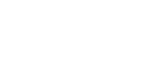1. Form the three inequalities that satisfy the unshaded region in the diagram below. (3 marks)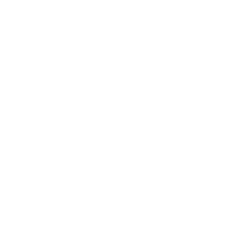1. Form the quadratic equation whose roots are x = -5/3 and x = 2.Give your answer in the form ax2 +bx +c =0 (2 marks)
1. Given that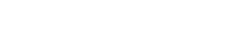Where a and b are rational numbers, find the values of a and b. (3marks)
1. Mwangi truncated 7/9 to 3 decimal places. Calculate the percentage error resulting from the truncating.     (3 marks)
1. Expand (1+2x)8 in ascending powers of up to and including the term in x8. Hence evaluate (1.02)8  (3 marks)
1. Log27(x + 5) – log27 (x – 3) = 2/3 (3 marks)
1.
1. Find the inverse of the matrix.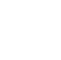(1 mark)
2. Hence determine the point of intersection of the lines.                           (2 marks)
y + x = 7
3x + y = 15
1. The first term of an arithmetic sequence is (2x+1) and the common difference is (x+1) if the product of the first and the second terms is zero, find the first three terms of the two possible sequences.  (4 marks)
1. Machine A can complete a piece of work in 6 hours while machine B can complete the same work in 10 hours. If both machines start working together and machine B breaks down after two hours, how long will it take machine A to complete the rest of the work. (3 marks)
1. An item that costs sh 24000 cash can be bought on hire purchase. A customer pays sh 6000 as deposit and then makes 6 monthly instalments of sh 3500 each. Calculate the monthly rate of compound interest, giving your answer to 1 d.p. (3 marks)
1. In the figure below CB is a tangent to the circle, DEB is a straight line DE:EB=1:3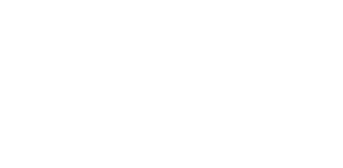Find BD and EB given that CB=8√3cm          (4 marks)
1. Make N the subject of the formula.    (3 marks)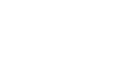1. An arc subtends an angle of 0.9 radians. If radius of circle is 13cm, find the length of the arc.    (3 marks)
1. The masses of 40 children being interviewed to join Std 1 by a certain school were as recorded in the table below.
 Mass(kg) 9 - 11 12 - 14 15 - 17 18 - 20 21 - 23 24 - 26 No of children 4 7 9 10 8 2

Calculate the mean mass of the students giving your answer to 4 S.F.                   (3 marks)
1. The equation of a circle is given by 2x2 + 8x + 2y2 – 10 = 0.   Determine the centre and the radius of the circle.  (3 marks)

SECTION II (50 MARKS)
Answer ONLY 5 Questions in this section in the spaces provided.

1. The figure below shows a triangle inscribed in a circle. AB = 6cm, BC = 9cm and AC = 10cm.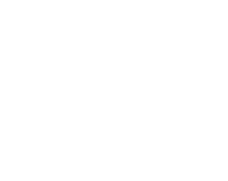Calculate
1. The interior angles of DABC.                                                                         (5 marks)
2. The radius of the circle.                                                                                  (2 marks)
3. The area of the shaded part.                                                                           (3 marks)
1. The table alongside shows the rates of taxation in a certain year.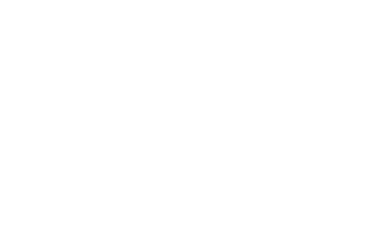In that year Mr. Kariuki a civil servant was earning a basic salary of Ksh. 27 000 per month. In addition he was entitled to other taxable allowances totalling to Ksh 11 000 per month and a personal relief of Ksh 1056 per month. He lives in a government house where he is paying a nominal rent of Ksh. 3 500 per month.
1. Calculate how much income tax Mr. Kariuki pays per month (in sh) (7 marks)
2. Kariuki’s other deductions per month were co-operative society contribution of sh 2500 and loan repayment of sh. 3000, calculate his net salary per month. (3 marks)
1. A group of young men decided to raise Ksh.480000 to start a business. Before actual payment was made four members pulled out and each of the remaining had to pay an additional Ksh.20000. Write an expression in terms of P for.
1.
1. Original contribution of each member.                                                           (1 mark)
2. Contribution after withdrawal of four members.                                           (1 mark)
2. Form an equation in P and hence determine the number of initial members.       (5 marks)
3. Three men Kamau, James and Hassan shared Shs.480000 such that Kamau: James is 3: 2 and James: Hassan is 4: 2. Find how much each got.    (3 marks)
1. Draw a cumulative frequency curve for the following data which shows the marks obtained by 80 form four students in a school. (5 marks)
 Marks 1-10 11-20 21-30 31-40 41-50 51-60 61-70 71-80 81-90 91-100 Frequency 3 5 5 9 11 15 14 8 6 4

1. The lower quartileQ1), median(Q2) and upper quartile(Q3) (3 marks)
2. The pass mark if 85% of these student are to pass (2 marks)
1. In chemistry form 4 classes, 1/3 of the class are girls and the rest boys, 4/5 of the boys and 9/10 of the girls are right handed while the rest are left handed. The probability that a right-handed student breaks a conical flask in any practical session is 3/10 and the corresponding probability of a left-handed student 4/10 . The probabilities are independent of the students gender.
1. Represent the above information on a tree diagram with independent probabilities. (2 marks)
2. Determine the probability that student chosen at random form the class is left handed and does not break a conical flask in simplest form.      (3 marks)
3. Determine the probability that a conical flask is broken in any chemistry practical session in simplest form.   (3 marks)
4. Determine the probability that a conical flask is not broken by a right-handed student in the simplest form.                                                                                             (2 marks)
1. Two straight lines and  intersect at a point P.Q and R are on the lines and  respectively such that PQ=5cm, PR=7cm and ÐRPQ=500
1. Draw the two lines.       (2 marks)
2. Using a ruler and pair of compasses only, draw a circle which is a tangent to line PR at R and passes through Q. (3 marks)
3. The line cuts the circle again at point S. Mark the point S and measure QS.     (2 marks)
4. Locate a point T on the minor arc such that QT<TS                                             (3 marks)
1. In the figure below, circle ABDF has centre O. CE is a tangent to the circle at D and AF = 12cm is the diameter, AB = BD and angle DAF = 27º.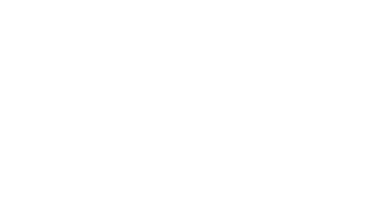1. Find the size of
2. Find the length of
2. BD.                                                                                                                 (4 marks)
1. ABCD is a quadrilateral with vertices A (3, 1), B (2, 4), C (4, 3), D (5, 1).
1. On the grid provided draw the image A¹B¹C¹D¹ image of ABCD under transformation matrix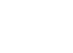and write down the co-ordinates.      (4 marks)
2. A transformation represented by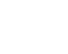maps A¹B¹C¹D¹ onto A¹¹B¹¹C¹¹D¹¹ determine the co-ordinates of the image and draw A¹¹B¹¹C¹¹D¹¹. (3 marks)
3. Determine the single matrix of transformation which maps ABCD onto A¹¹B¹¹C¹¹D¹¹ and describe the transformation fully.                                                (3 marks)## MARKING SCHEME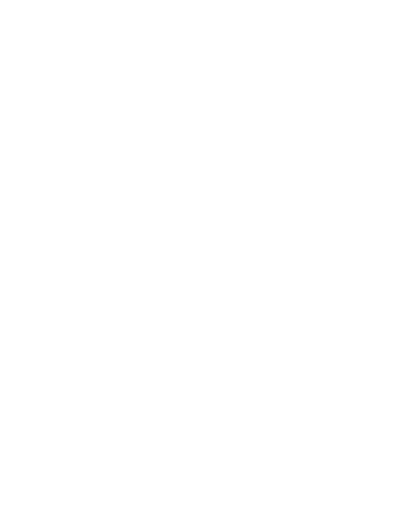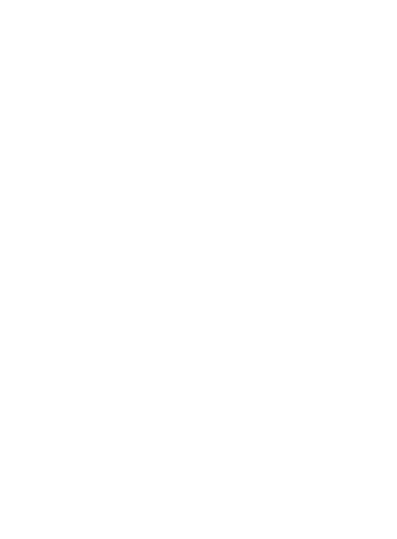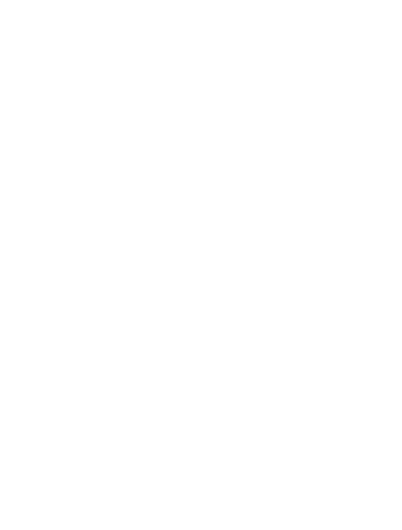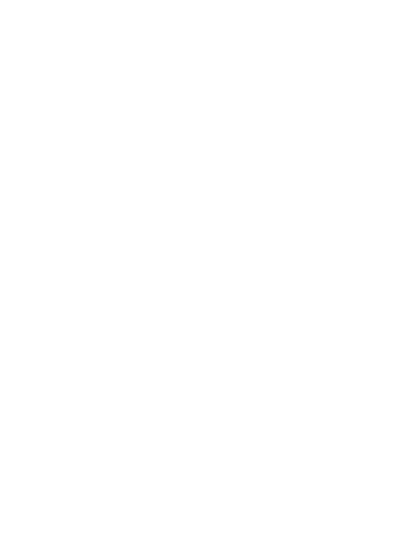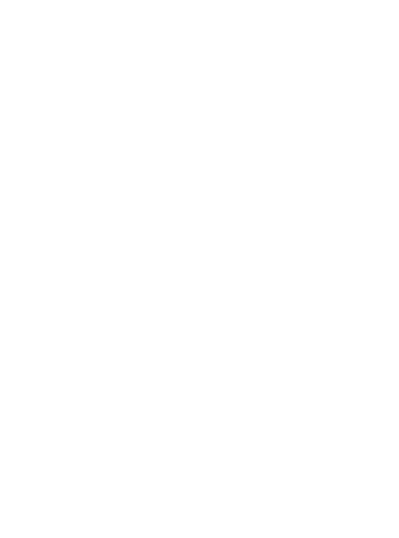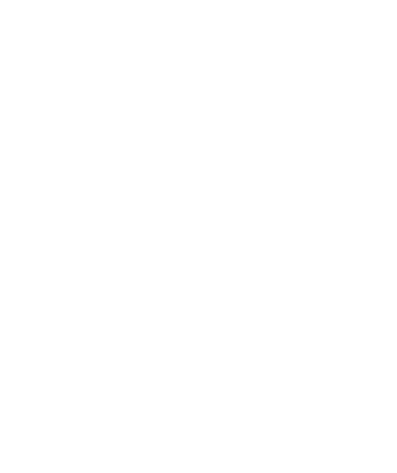#### Download MATHEMATICS PAPER 2 - KCSE 2019 NYANDARUA PRE MOCK EXAMINATION.

• ✔ To read offline at any time.
• ✔ To Print at your convenience
• ✔ Share Easily with Friends / Students